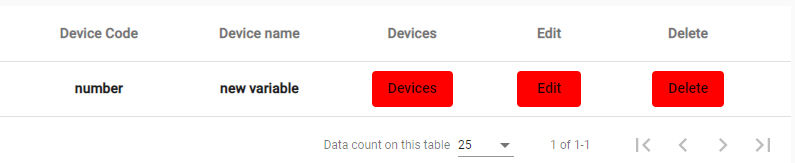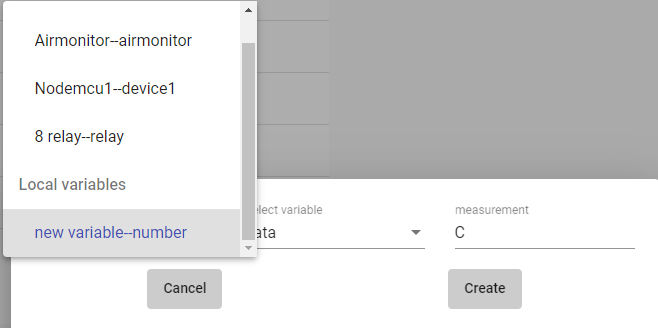top of page
Search

# 15-Local VariableLocal variables allow us to create new variables by making calculations using our data from the devices.

We can use these variables in our instrument panels and alarm systems.

You can also define fixed values ​​that you want to use in calculations or display on dashboards here.

We can reach the setting page by clicking the local variables button from the main menu.Local variable name : the name of the variable we will create

Local variable code : variable codeWe can set the content of the variable with the Devices button.

If we want to create a new variable by taking data from the devices and calculating, we can select the variables with the add device button.Select device : Here is the device list we added to the system. We can select the device we want to use from the list.

Select variable: After device selection; device-related variables are listed here. Let's choose the variable we will use in the formula

Formula code : We need to create a code to write the formula more easily. We give the code x to the variable.In this formula, we will create the new variable by adding 10.5 to the temperature value.

You can use multiplication(*), division(/) , addition(+), subtraction(-), and parentheses(). For fractional numbers we should use dot(.).

We write x + 10.5 in the Math formula section.

We save our variable with Update the formula.Now we can use this variable in the dashboard.